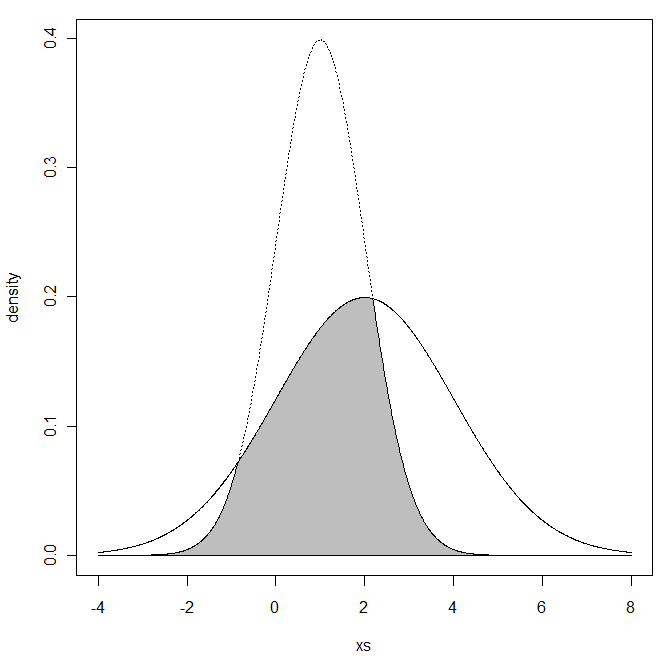Want to share your content on R-bloggers? click here if you have a blog, or here if you don't.

I’m currently working with a client who is researching best covariance matrices for financial time series.  Specifically, they are looking at what best describes 1 month out of sample distributions.  They are not concerned with the means, just the variance.

A paper on the subject from the Journal of Portfolio Management — “A Test of Covariance Matrix Forecasting Methods” by Zakamulin.

The test boils down to the sum of the squares of the differences in the upper half of the covariance matrices.  This is really just the Frobenius Norm of the difference between the matrices.

My issue with the Frobenius Norm has always been it is not, directly, a measure of the similarity between two distributions.  If we are using an estimated matrix to make investing decisions based on the distribution of likely outcomes, shouldn’t we concern ourselves with the degree to which our estimated matrix can predict the distribution of outcomes?

Some thinking, noodling with code, thinking some more, and then a computer forced reboot (hosing said code) got me to actually Google the subject.  First thing that came up was this Cross Validated post.  Also this helpful presentation.  Seems what I was doing had been invented before (like that’s a surprise).

“The Overlapping Coefficient (OVL) refers to the area under the two probability density functions simultaneously.”(Graph pulled from that Cross Validated post)

OVL is defined on the range of (0,1].  1 is a perfect fit with lower numbers a worse fit.

So how does the OVL compare to the Frobenius Norm (FN)?  When will their preferences in matrices differ?

We will make use of the R code from Cross Validated and use a univariate example as it is easy to visualize.  We will compare two distributions to a N(0,0.01) variable.  In the graph, the N(0,0.01) value is the solid line.  The distribution to compare is the dotted.

Full code at the end.

In the univariate case, FN is

FN is on the range of [0,∞).  0 is a perfect fit with higher numbers a worse fit.

Case 1:
X1 ~ N(0,0.01) vs X2 ~ N(0,0.0225)  — Standard Deviation of 0.1 vs 0.15.

OVL = 0.8064
FN = 0.0125

Case 2:
X1 ~ N(0,0.01) vs X2 ~ N(0,0.0025)  — Standard Deviation of 0.1 vs 0.05.
OVL = 0.6773
FN = 0.0075

The two competing distributions are both 0.05 different from the true standard deviation.  The OVL statistic prefers the Case 1 contender.  FN prefers the Case 2.

Will the nature of the statistics cause the OVL to prefer higher SD values with the FN to prefer lower SD values?

Text in RED is the Standard Deviation being compared.

In general, we see the trend from upper left to lower right.  These statistics correlate on how they rank distributions in the way we expect.  The will both always pick the same preference on adjacent points.

We see that FN punishes over estimation of the SD.  That is, the higher numbers in red extend in a flatter arch down and to the right.

We see that OVL punishes under estimation of the SD.  That is the lower numbers in red extend in a steep arch down and to the right.

The FN is approximately indifferent between SD=0.02 and SD=0.14.  OVL would pick the 0.14 value.

The OVL is approximately indifferent between SD=0.07 and SD=0.14.  FN would pick the 0.07 value.

This leaves me unable to say which is better.  Intuitively, I prefer the OVL.  In a later post, I will develop a multivariate version of OVL using numerical integration.

As promised, R code for the above (yet again, hat tip to Cross Validated for a large portion of this):
min.f1f2 <- function(x, mu1, mu2, sd1, sd2) {
f1 <- dnorm(x, mean=mu1, sd=sd1)
f2 <- dnorm(x, mean=mu2, sd=sd2)
pmin(f1, f2)
}

#CASE 1
mu1 <- 0
mu2 <- 0
sd1 <- .1
sd2 <- .15

xs <- seq(min(mu1 - 3*sd1, mu2 - 3*sd2), max(mu1 + 3*sd1, mu2 + 3*sd2), .01)
f1 <- dnorm(xs, mean=mu1, sd=sd1)
f2 <- dnorm(xs, mean=mu2, sd=sd2)

plot(xs, f1, type=”l”, ylim=c(0, max(f1,f2)), ylab=”density”)
lines(xs, f2, lty=”dotted”)
ys <- min.f1f2(xs, mu1=mu1, mu2=mu2, sd1=sd1, sd2=sd2)
xs <- c(xs, xs)
ys <- c(ys, ys)
polygon(xs, ys, col=”gray”)

print(paste(“OVL:”,integrate(min.f1f2, -Inf, Inf, mu1=mu1, mu2=mu2, sd1=sd1, sd2=sd2)\$value,sep=” “))
print(paste(“FN:”,abs(sd1^2 – sd2^2),sep=” “))

#CASE 2
mu1 <- 0
mu2 <- 0
sd1 <- .1
sd2 <- .05

xs <- seq(min(mu1 - 3*sd1, mu2 - 3*sd2), max(mu1 + 3*sd1, mu2 + 3*sd2), .01)
f1 <- dnorm(xs, mean=mu1, sd=sd1)
f2 <- dnorm(xs, mean=mu2, sd=sd2)

plot(xs, f1, type=”l”, ylim=c(0, max(f1,f2)), ylab=”density”)
lines(xs, f2, lty=”dotted”)
ys <- min.f1f2(xs, mu1=mu1, mu2=mu2, sd1=sd1, sd2=sd2)
xs <- c(xs, xs)
ys <- c(ys, ys)
polygon(xs, ys, col=”gray”)

print(paste(“OVL:”,integrate(min.f1f2, -Inf, Inf, mu1=mu1, mu2=mu2, sd1=sd1, sd2=sd2)\$value,sep=” “))
print(paste(“FN:”,abs(sd1^2 – sd2^2),sep=” “))

#Compare over range of SD values
x = matrix(seq(from=.01,to=.2,by=.01))
fn = apply(x,1,function(s1) abs(.01 – s1^2))
ovl = apply(x,1,function(s1) integrate(min.f1f2, -Inf, Inf, mu1=0, mu2=0, sd1=s1, sd2=0.1)\$value)

toGraph = cbind(x,fn,ovl)

#indiffernce compared to SD=0.14
fn = abs(0.01 – .14^2)
ovl = integrate(min.f1f2, -Inf, Inf, mu1=0, mu2=0, sd1=.14, sd2=0.1)\$value

plot(toGraph[,2],toGraph[,3],xlab=”FN”,ylab=”OVL”,ylim=c(0,1.1))
abline(h=ovl,lty=”dotted”)
abline(v=fn,lty=”dotted”)
text(toGraph[,2],toGraph[,3],toGraph[,1],col=”red”,pos=1)